The Principle of Relativity: A Collection of Original Memoirs on the Special and General Theory of Relativity

Contents:
Author: Albert Einstein  | Date: 1911

W. Perrett and G.B. Jeffery Physics

# On the Influence of Gravitation on the Propagation of Light

A. EINSTEIN

Translated from "Über den Einfluss der Schwerkraft auf die Ausbreitung des Lichtes," Annalen der Physik, 35, 1911.

# On the Influence of Gravitation on the Propagation of Light

BY A. EINSTEIN

IN a memoir published four years ago* I tried to answer the question whether the propagation of light is influenced by gravitation. I return to this theme, because my previous presentation of the subject does not satisfy me, and for a stronger reason, because I now see that one of the most important consequences of my former treatment is capable of being tested experimentally. For it follows from the theory here to be brought forward, that rays of light, passing close to the sun, are deflected by its gravitational field, so that the angular distance between the sun and a fixed star appearing near to it is apparently increased by nearly a second of arc.

In the course of these reflexions further results are yielded which relate to gravitation. But as the exposition of the entire group of considerations would be rather difficult to follow, only a few quite elementary reflexions will be given in the following pages, from which the reader will readily be able to inform himself as to the suppositions of the theory and its line of thought. The relations here deduced, even if the theoretical foundation is sound, are valid only to a first approximation.

### § 1. A Hypothesis as to the Physical Nature of the Gravitational Field

In a homogeneous gravitational field (acceleration of gravity γ) let there be a stationary system of co-ordinates K, orientated so that the lines of force of the gravitational field run in the negative direction of the axis of z. In a space free of gravitational fields let there be a second system of coordinates K′, moving with uniform acceleration (γ) in the positive direction of its axis of z. To avoid unnecessary complications, let us for the present disregard the theory of relativity, and regard both systems from the customary point of view of kinematics, and the movements occurring in them from that of ordinary mechanics.

Relatively to K, as well as relatively to K′, material points which are not subjected to the action of other material points, move in keeping with the equations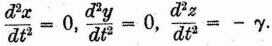For the accelerated system K′ this follows directly from Galileo’s principle, but for the system K, at rest in a homogeneous gravitational field, from the experience that all bodies in such a field are equally and uniformly accelerated. This experience, of the equal falling of all bodies in the gravitational field, is one of the most universal which the observation of nature has yielded; but in spite of that the law has not found any place in the foundations of our edifice of the physical universe.

But we arrive at a very satisfactory interpretation of this law of experience, if we assume that the systems K and K′ are physically exactly equivalent, that is, if we assume that we may just as well regard the system K as being in a space free from gravitational fields, if we then regard K as uniformly accelerated. This assumption of exact physical equivalence makes it impossible for us to speak of the absolute acceleration of the system of reference, just as the usual theory of relativity forbids us to talk of the absolute velocity of a system;* and it makes the equal falling of all bodies in a gravitational field seem a matter of course.

As long as we restrict ourselves to purely mechanical processes in the realm where Newton’s mechanics holds sway, we are certain of the equivalence of the systems K and K′.

But this view of ours will not have any deeper significance unless the systems K and K′ are equivalent with respect to all physical processes, that is, unless the laws of nature with respect to K are in entire agreement with those with respect to K′. By assuming this to be so, we arrive at a principle which, if it is really true, has great heuristic importance. For by theoretical consideration of processes which take place relatively to a system of reference with uniform acceleration, we obtain information as to the career of processes in a homogeneous gravitational field. We shall now show, first of all, from the standpoint of the ordinary theory of relativity, what degree of probability is inherent in our hypothesis.

### § 2. On the Gravitation of Energy

One result yielded by the theory of relativity is that the inertia mass of a body increases with the energy it contains; if the increase of energy amounts to E, the increase in inertia mass is equal to E/c2, when c denotes the velocity of light. Now is there an increase of gravitating mass corresponding to this increase of inertia mass? If not, then a body would fall in the same gravitational field with varying acceleration according to the energy it contained. That highly satisfactory result of the theory of relativity by which the law of the conservation of mass is merged in the law of conservation of energy could not be maintained, because it would compel us to abandon the law of the conservation of mass in its old form for inertia mass, and maintain it for gravitating mass.

But this must be regarded as very improbable. On the other hand, the usual theory of relativity does not provide us with any argument from which to infer that the weight of a body depends on the energy contained in it. But we shall show that our hypothesis of the equivalence of the systems K and K′ gives us gravitation of energy as a necessary consequence.

Let the two material systems S1 and S2, provided withinstruments of measurement, be situated on the z-axis of K at the distance h from each other,* so that the gravitation potential in S2 is greater than that in S1 by γh. Let a definite quantity of energy E be emitted from S2 towards S1. Let the quantities of energy in S1 and S2 be measured by contrivances which— brought to one place in the system z and there compared—shall be perfectly alike. As to the process of this conveyance of energy by radiation we can make no a priori assertion, because we do not know the influence of the gravitational field on the radiation and the measuring instruments in S1 and S2.

But by our postulate of the equivalence of K and K′ we are able, in place of the system K in a homogeneous gravitational field, to set the gravitation-free system K′, which moves with uniform acceleration in the direction of positive z, and with the z-axis of which the material systems S1 and S2 are rigidly connected.

We judge of the process of the transference of energy by radiation from S2 to S1 from a system K0, which is to be free from acceleration. At the moment when the radiation energy E2 is emitted from S2 toward S1, let the velocity of K′ relatively to K0 be zero. The radiation will arrive at S1 when the time h/c has elapsed (to a first approximation). But at this moment the velocity of S1 relatively to K0 is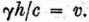Therefore by the ordinary theory of relativity the radiation arriving at S1 does not possess the energy E2, but a greater energy E1, which is related to E2 to a first approximation by the equation*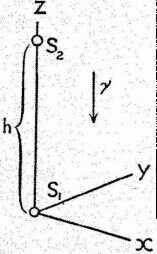FIG. 5.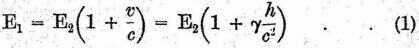By our assumption exactly the same relation holds if the same process takes place in the system K, which is not accelerated, but is provided with a gravitational field. In this case we may replace γh by the potential Φ of the gravitation vector in S2, if the arbitrary constant of Φ in S1 is equated to zero. We then have the equation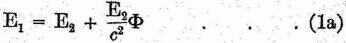This equation expresses the law of energy for the process under observation. The energy E1 arriving at S1 is greater than the energy E2, measured by the same means, which was emitted in S2, the excess being the potential energy of the mass E2/c2 in the gravitational field. It thus proves that for the fulfilment of the principle of energy we have to ascribe to the energy E, before its emission in S2, a potential energy due to gravity, which corresponds to the gravitational mass E/c2. Our assumption of the equivalence of K and K′ thus removes the difficulty mentioned at the beginning of this paragraph which is left unsolved by the ordinary theory of relativity.

The meaning of this result is shown particularly clearly if we consider the following cycle of operations:—

1. The energy E, as measured in S2, is emitted in the form of radiation in S2 towards S1, where, by the result just obtained, the energy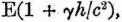as measured in S1, is absorbed.

2. A body W of mass M is lowered from S2 to S1, work Mγh being done in the process.

3. The energy E is transferred from S1 to the body W while W is in S1. Let the gravitational mass M be thereby changed so that it acquires the value M′.

4. Let W be again raised to S2, work M′γh being done in the process.

5. Let E be transferred from W back to S2.

The effect of this cycle is simply that S1 has undergone the increase of energy Eγh/c2, and that the quantity of energy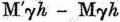has been conveyed to the system in the form of mechanical work. By the principle of energy, we must therefore have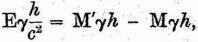or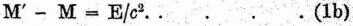The increase in gravitational mass is thus equal to E/c2, and therefore equal to the increase in inertia mass as given by the theory of relativity.

The result emerges still more directly from the equivalence of the systems K and K′, according to which the gravitational mass in respect of K is exactly equal to the inertia mass in respect of K′; energy must therefore possess a gravitational mass which is equal to its inertia mass. If a mass M0 be suspended on a spring balance in the system K′, the balance will indicate the apparent weight M0γ on account of the inertia of M0. If the quantity of energy E be transferred to M0, the spring balance, by the law of the inertia of energy, will indicate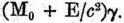By reason of our fundamental assumption exactly the same thing must occur when the experiment is repeated in the system K, that is, in the gravitational field.

### § 3. Time and the Velocity of Light in the Gravitational Field

If the radiation emitted in the uniformly accelerated system K′ in S2 toward S1 had the frequency ν2 relatively to the clock in S2, then, relatively to S1, at its arrival in S1 it no longer has the frequency ν2 relatively to an identical clock in S1, but a greater frequency ν1, such that to a first approximation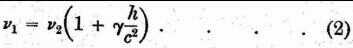For if we again introduce the unaccelerated system of reference K0, relatively to which, at the time of the emission of light, K′ has no velocity, then S1, at the time of arrival of the radiation at S1, has, relatively to K0, the velocity γh/c, from which, by Doppler’s principle, the relation as given results immediately.

In agreement with our assumption of the equivalence of the systems K′ and K, this equation also holds for the stationary system of co-ordinates K, provided with a uniform gravitational field, if in it the transference by radiation takes place as described. It follows, then, that a ray of light emitted in S2 with a definite gravitational potential, and possessing at its emission the frequency ν2—compared with a clock in S2—will, at its arrival in S1, possess a different frequency ν1—measured by an identical clock in S1. For γh we substitute the gravitational potential Φ of S2—that of S1

being taken as zero—and assume that the relation which we have deduced for the homogeneous gravitational field also holds for other forms of field. Then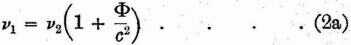This result (which by our deduction is valid to a first approximation) permits, in the first place, of the following application. Let ν0 be the vibration-number of an elementary light-generator, measured by a delicate clock at the same place. Let us imagine them both at a place on the surface of the Sun (where our S2 is located). Of the light there emitted, a portion reaches the Earth (S1), where we measure the frequency of the arriving light with a clock U in all respects resembling the one just mentioned. Then by (2a),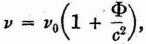where Φ is the (negative) difference of gravitational potential between the surface of the Sun and the Earth. Thus according to our view the spectral lines of sunlight, as compared with the corresponding spectral lines of terrestrial sources of light, must be somewhat displaced toward the red, in fact by the relative amount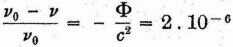If the conditions under which the solar bands arise were exactly known, this shifting would be susceptible of measurement. But as other influences (pressure, temperature) affect the position of the centres of the spectral lines, it is difficult to discover whether the inferred influence of the gravitational potential really exists.*

On a superficial consideration equation (2), or (2a), respectively, seems to assert an absurdity. If there is constant transmission of light from S2 to S1, how can any other number of periods per second arrive in S1 than is emitted in S2? But the answer is simple. We cannot regard ν2 or respectively ν1 simply as frequencies (as the number of periods per second) since we have not yet determined the time in system K. What ν2 denotes is the number of periods with reference to the time-unit of the clock U in S2, while ν1 denotes the number of periods per second with reference to the identical clock in S1. Nothing compels us to assume that the clocks U in different gravitation potentials must be regarded as going at the same rate. On the contrary, we must certainly define the time in K in such a way that the number of wave crests and troughs between S2 and S1 is independent of the absolute value of time; for the process under observation is by nature a stationary one. If we did not satisfy this condition, we should arrive at a definition of time by the application of which time would merge explicitly into the laws of nature, and this would certainly be unnatural and unpractical. Therefore the two clocks in S1 and S2 do not both give the "time" correctly. If we measure time in S1 with the clock U, then we must measure time in S2 with a clock which goes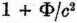times more slowly than the clock U when compared with U at one and the same place. For when measured by such a clock the frequency of the ray of light which is considered above is at its emission in S2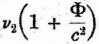and is therefore, by (2a), equal to the frequency ν1 of the same ray of light on its arrival in S1.

This has a consequence which is of fundamental importance for our theory. For if we measure the velocity of light at different places in the accelerated, gravitation-free system K′, employing clocks U of identical constitution, we obtain the same magnitude at all these places. The same holds good, by our fundamental assumption, for the system K as well. But from what has just been said we must use clocks of unlike constitution, for measuring time at places with differing gravitation potential. For measuring time at a place which, relatively to the orion of the co-ordinates, has the gravitation potential Φ, we must employ a clock which— when removed to the origin of co-ordinates—goes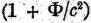times more slowly than the clock used for measuring time at the origin of co-ordinates. If we call the velocity of light at the origin of co-ordinates c0, then the velocity of light c at a place with the gravitation potential Φ will be given by the relation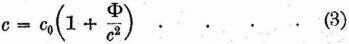The principle of the constancy of the velocity of light holds good according to this theory in a different form from that which usually underlies the ordinary theory of relativity.

### § 4. Bending of Light-Rays in the Gravitational Field

From the proposition which has just been proved, that the velocity of light in the gravitational field is a function of the place, we may easily infer, by means of Huyghens’s principle, that light-rays propagated across a gravitational field undergo deflexion. For let E be a wave front of a plane light-wave at the time t, and let P1 and P2 be two points in that plane at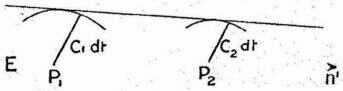FIG. 6.

unit distance from each other. P1 and P2 lie in the plane of the paper, which is chosen so that the differential coefficient of Φ, taken in the direction of the normal to the plane, vanishes, and therefore also that of c. We obtain the corresponding wave front at time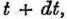or, rather, its line of section with the plane of the paper, by describing circles round the points P1 and P2 with radii c1dt and c2dt respectively, where c1 and c2 denote the velocity of light at the points P1 and P2 respectively, and by drawing the tangent to these circles. The angle through which the light-ray is deflected in the path cdt is therefore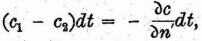if we calculate the angle positively when the ray is bent toward the side of increasing n′. The angle of deflexion per unit of path of the light-ray is thus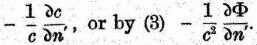Finally, we obtain for the deflexion which a light-ray experiences toward the side n′ on any path (s) the expression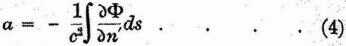We might have obtained the same result by directly considering the propagation of a ray of light in the uniformly accelerated system K′, and transferring the result to the system K, and thence to the case of a gravitational field of any form.

By equation (4) a ray of light passing along by a heavenly body suffers a deflexion to the side of the diminishing gravitational potential, that is, on the side directed toward the heavenly body, of the magnitude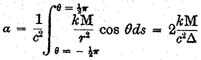where k denotes the constant of gravitation, M the mass of the heavenly body, Δ the distance of the ray from the centre of the body. A ray of light going past the Sun would accordingly undergo deflexion to the amount of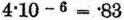seconds of arc. The angular distance of the star from the centre of the Sun appears to be increased by this amount. As the fixed stars in the parts of the sky near the Sun are visible during total eclipses of the Sun, this consequence of the theory may be compared with experience. With the planet Jupiter the displacement to be expected reaches to about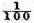of the amount given. It would be a most desirable thing if astronomers would take up the question here raised. For apart from any theory there is the question whether it is possible with the equipment at present available to detect an influence of gravitational fields on the propagation of light.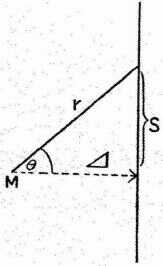FIG. 7.

* A. Einstein, Jahrbuch für Radioakt. und Elektronik, 4, 1907.

* Of course we cannot replace any arbitrary gravitational field by a state of motion of the system without a gravitational field, any more than, by a transformation of relativity, we can transform all points of a medium in any kind of motion to rest.

* The dimensions of S1 and S2 are regarded as infinitely small in comparison with h.

* See above, pp. 69–71.

* L. F. Jewell (Journ. de Phys., 6, 1897, p. 84) and particularly Ch. Fabry and H. Boisson (Comptes rendus, 148, 1909, pp. 688–690) have actually found such displacements of fine spectral lines toward the red end of the spectrum, of the order of magnitude here calculated, but have ascribed them to an effect of pressure in the absorbing layer.

Contents:

### Related Resources

Albert Einstein
Classics of Non-fiction

Title: The Principle of Relativity: A Collection of Original Memoirs on the Special and General Theory of Relativity

Select an option:

## Email Options

Title: The Principle of Relativity: A Collection of Original Memoirs on the Special and General Theory of Relativity

Select an option: